GeeksforGeeks App
Open AppBrowser
Continue

# Data Visualization using Plotnine and ggplot2 in Python

Data Visualization is the technique of presenting data in the form of graphs, charts, or plots. Visualizing data makes it easier for the data analysts to analyze the trends or patterns that may be present in the data as it summarizes the huge amount of data in a simple and easy-to-understand format.

In this article, we will discuss how to visualize data using plotnine in Python which is a strict implementation of the grammar of graphics. Before starting let’s understand a brief about what is the grammar of graphics.

## What is the Grammar of Graphics?

A grammar of graphics is basically a tool that enables us to describe the components of a given graphic. Basically, this allows us to see beyond the named graphics, (scatter plot, to name one) and to basically see the underlying statistics behind it. Consider grammar of graphics as the grammar of English where we use different words, tenses, punctuations to form a sentence.

## Components of Grammar of graphics

Typically, to build or describe any visualization with one or more dimensions, we can use the components shown in the below image.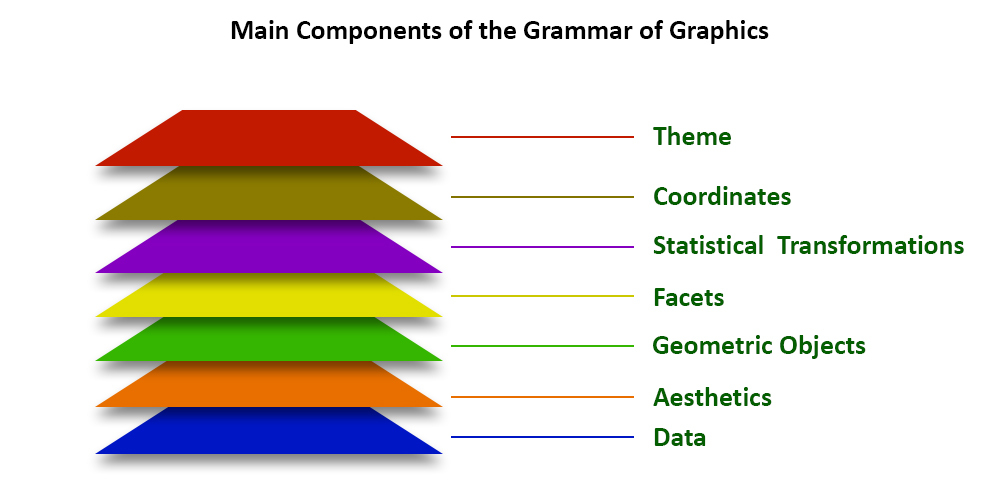First, we will see the three main components that are required to create a plot, and without these components, the plotnine would not be able to plot the graph. These are-

• Data is the dataset that is used for plotting the plot.
• Aesthetics (aes) is the mapping between the data variables and the variables used by the plot such as x-axis, y-axis, color, fill, size, labels, alpha, shape, line width, line type.
• Geometric Objects (geoms) is the type of plot or a geometric object that we want to use such as point, line, histogram, bar, boxplot, etc.

There are various optional components that can make the plot more meaningful and presentable. These are –

• Facets allow the data to be divided into groups and each group is plotted separately.
• Statistical transformations compute the data before plotting it.
• Coordinates define the position of the object in a 2D plane.
• Themes define the presentation of the data such as font, color, etc.

## Installation

The plotnine is based on ggplot2 in R Programming language which is used to implement grammar of graphics in Python. To install plotnine type the below command in the terminal.

`pip install plotnine`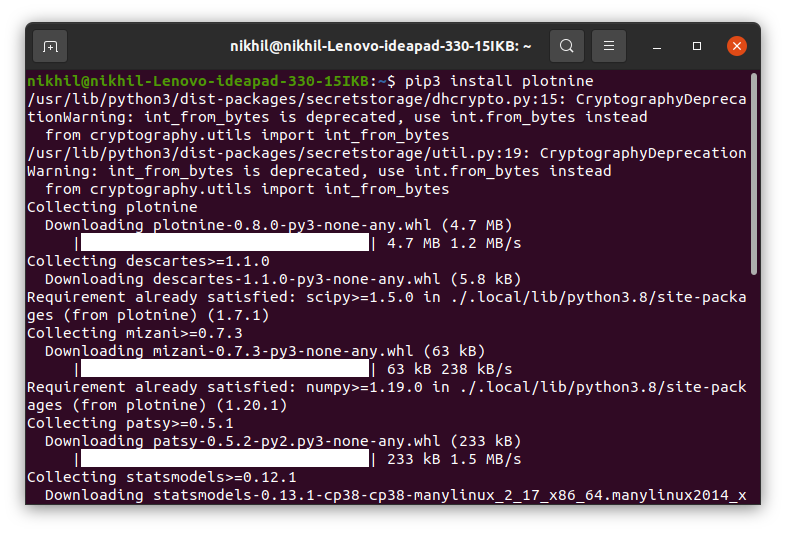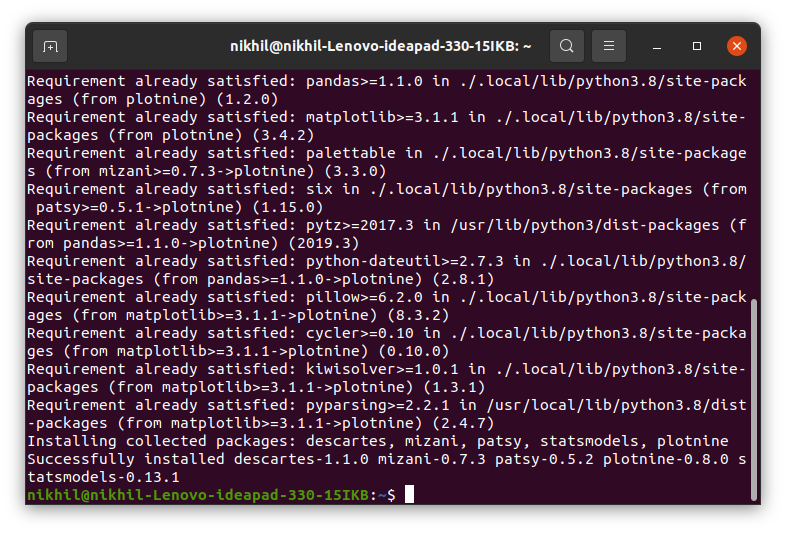## Plotting Data using Plotnine and ggplot in Python

Here we will use the three main components i.e. data, aesthetics, and geometric objects for plotting our data. Let’s go through each component in detail.

### Data

The data is the dataset which is needed to be plotted. We can specify the data using the ggplot constructor and passing the dataset to that constructor.

### Example: Specifying dataset for the ggplot

We will use the Iris dataset and will read it using Pandas.

## Python3

 `import` `pandas as pd``from` `plotnine ``import` `ggplot`` ` `# reading dataset``df ``=` `pandas.read_csv(``"Iris.csv"``)`` ` `# passing the data to the ggplot ``# constructor``ggplot(df)`

Output: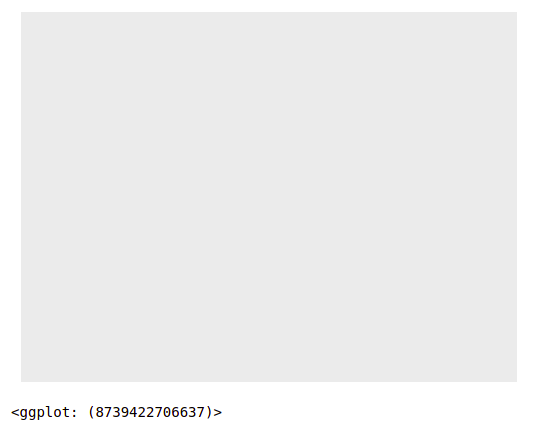This will give us a blank output as we have not specified the other two main components.

### Aesthetics

Now let’s define the variable that we want to use for each axis in the plot. Aesthetics maps data variables to graphical attributes, like 2D position and color.

## Python3

 `import` `pandas as pd``from` `plotnine ``import` `ggplot, aes`` ` `# reading dataset``df ``=` `pd.read_csv(``"Iris.csv"``)`` ` `ggplot(df) ``+` `aes(x``=``"Species"``, y``=``"SepalLengthCm"``)`

Output: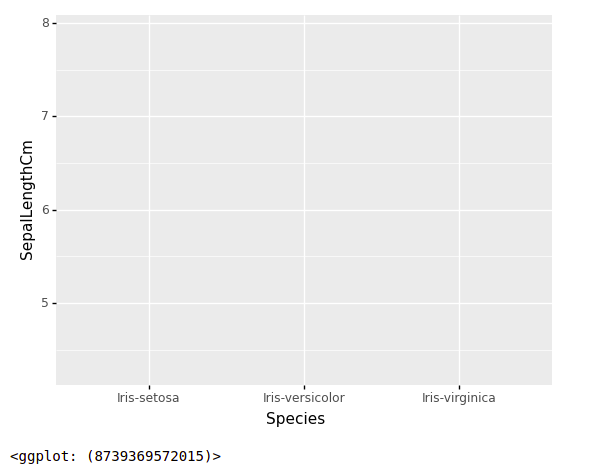In the above example, we can see that Species is shown on the x-axis and sepal length is shown on the y-axis. But still there is no figure in the plot. This can be added using geometric objects.

### Geometric Objects

After defining the data and the aesthetics we need to define the type of plot that we want for visualization. This tells the plotline that how the data points should be shown. It provides a variety of geometric objects like scatter plots, line charts, bar charts, box plots, etc. Let’s see a variety of them and how to use them.

Note: For the list of all the geoms refer to the plotnine’s geom API reference.

## Python3

 `import` `pandas as pd``from` `plotnine ``import` `ggplot, aes, geom_col`` ` `# reading dataset``df ``=` `pd.read_csv(``"Iris.csv"``)`` ` `ggplot(df) ``+` `aes(x``=``"Species"``, y``=``"SepalLengthCm"``) ``+` `geom_col()`

Output: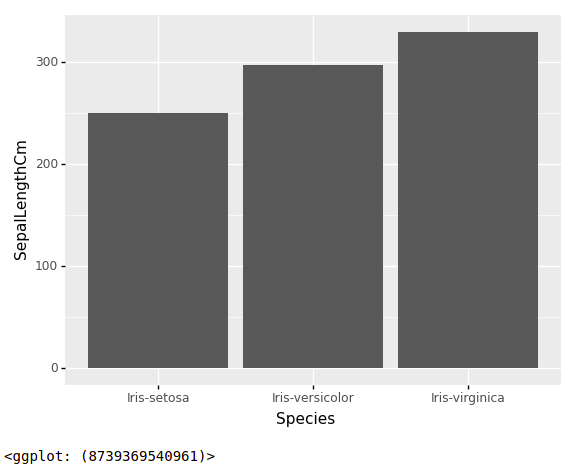In the above example, we have used the geam_col() geom that is a bar plot with the base on the x-axis. We can change this to different types of geoms that we find suitable for our plot.

## Python3

 `import` `pandas as pd``from` `plotnine ``import` `ggplot, aes, geom_histogram`` ` `# reading dataset``df ``=` `pd.read_csv(``"Iris.csv"``)`` ` `ggplot(df) ``+` `aes(x``=``"SepalLengthCm"``) ``+` `geom_histogram()`

Output: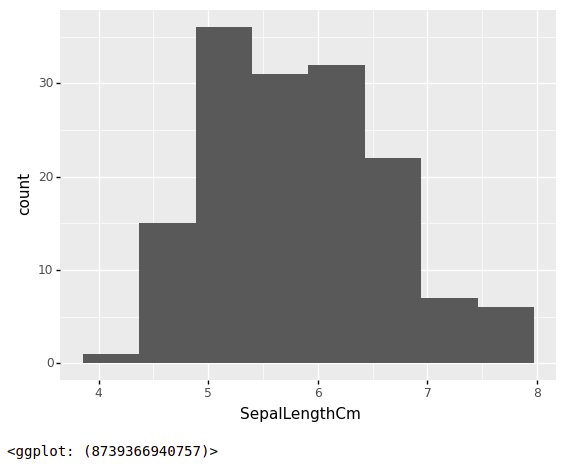## Python3

 `import` `pandas as pd``from` `plotnine ``import` `ggplot, aes, geom_point`` ` `# reading dataset``df ``=` `pd.read_csv(``"Iris.csv"``)`` ` `ggplot(df) ``+` `aes(x``=``"Species"``, y``=``"SepalLengthCm"``) ``+` `geom_point()`

Output: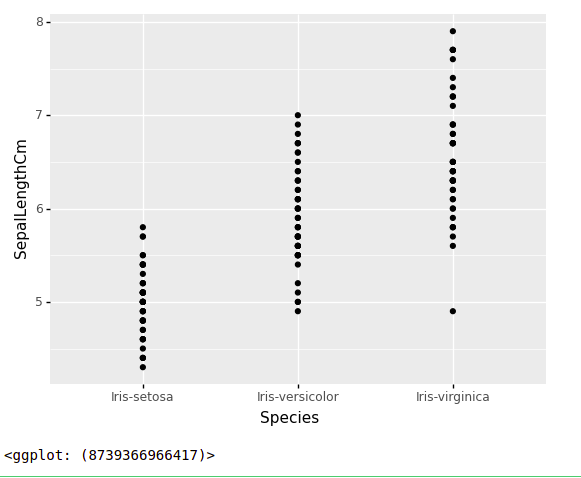## Python3

 `import` `pandas as pd``from` `plotnine ``import` `ggplot, aes, geom_boxplot`` ` `# reading dataset``df ``=` `pd.read_csv(``"Iris.csv"``)`` ` `# passing the data to the ggplot ``# constructor``ggplot(df) ``+` `aes(x``=``"Species"``, y``=``"SepalLengthCm"``) ``+` `geom_boxplot()`

Output: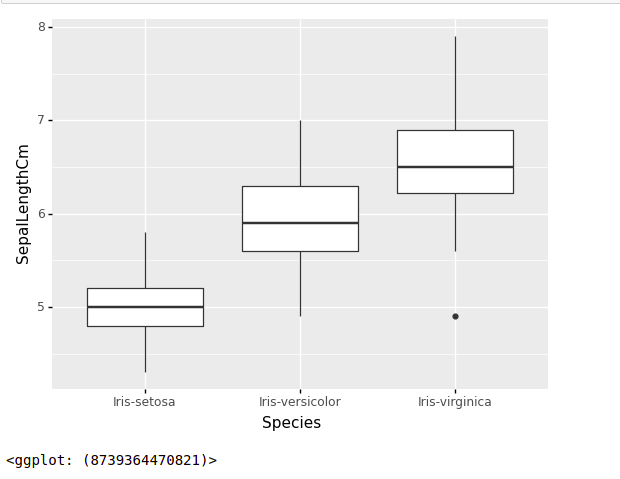## Python3

 `import` `pandas as pd``from` `plotnine ``import` `ggplot, aes, geom_line`` ` `# reading dataset``df ``=` `pd.read_csv(``"Iris.csv"``)`` ` `ggplot(df) ``+` `aes(x``=``"Species"``, y``=``"SepalLengthCm"``) ``+` `geom_line()`

Output: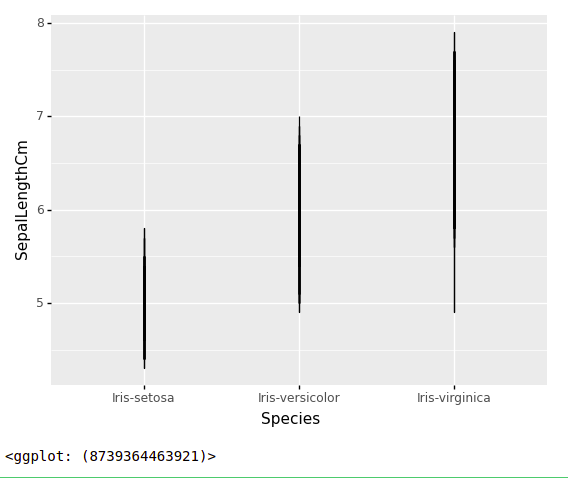Till now we have learnt about how to create a basic chart using the concept of grammar of graphics and it’s three main components. Now let’s learn how to customize these charts using the other optional components.

## Enhacing Data visualizations using plotnine and ggplot

Here we will learn about the remaining optional components. These components are –

• Facets
• Statistical transformations
• Coordinates
• Themes

### Facets

Facets are used to plot subsets of data. it allows an individual plot for groups of data in the same image.

For example, let’s consider the tips dataset that contains information about people who probably had food at a restaurant and whether or not they left a tip, their age, gender and so on. Lets have a look at it.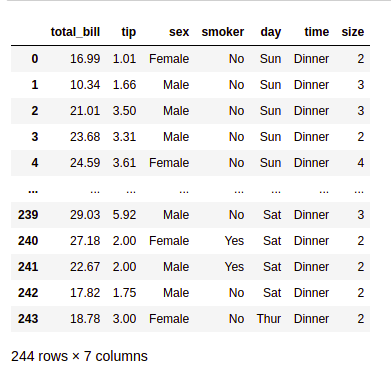Now let’s suppose we want to plot about what was the total bill according to the gender and on each day. In such cases facets can be very useful, let’s see how.

## Python3

 `import` `pandas as pd``from` `plotnine ``import` `ggplot, aes, facet_grid, labs, geom_col`` ` `# reading dataset``df ``=` `pd.read_csv(``"tips.csv"``)`` ` `(``    ``ggplot(df)``    ``+` `facet_grid(facets``=``"~sex"``)``    ``+` `aes(x``=``"day"``, y``=``"total_bill"``)``    ``+` `labs(``        ``x``=``"day"``,``        ``y``=``"total_bill"``,``    ``)``    ``+` `geom_col()``)`

Output: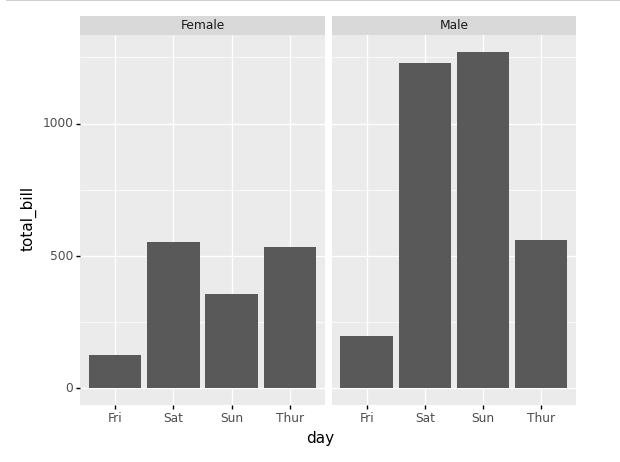### Statistical transformations

Statistical transformations means computing data before plotting it. It can be seen in the case of a histogram. Now let’s consider the above example, where we wanted to find the measurement of the sepal length column and now we want to distribute that measurement into 15 columns. The geom_histogram() function of the plotnine computes and plot this data automatically.

## Python3

 `import` `pandas as pd``from` `plotnine ``import` `ggplot, aes, geom_histogram`` ` `# reading dataset``df ``=` `pd.read_csv(``"Iris.csv"``)`` ` `ggplot(df) ``+` `aes(x``=``"SepalLengthCm"``) ``+` `geom_histogram(bins``=``15``)`

Output: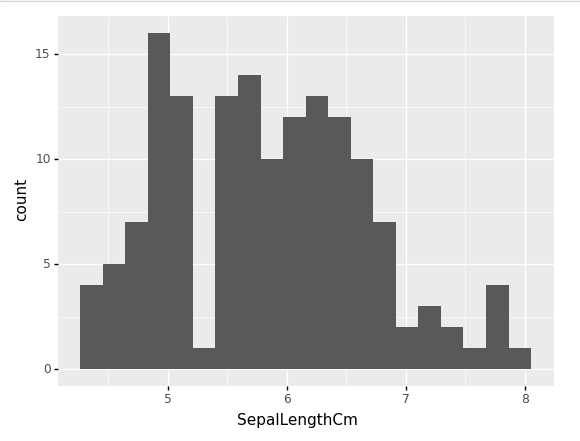### Coordinates

The coordinates system defines the imappinof the data point with the 2D graphical location on the plot. Let’s see the above example of histogram, we want to plot this histogram horizontally. We can simply do this by using the coord_flip() function.

## Python3

 `import` `pandas as pd``from` `plotnine ``import` `ggplot, aes, geom_histogram, coord_flip`` ` `# reading dataset``df ``=` `pd.read_csv(``"Iris.csv"``)`` ` `(``    ``ggplot(df)``    ``+` `aes(x``=``"SepalLengthCm"``)``    ``+` `geom_histogram(bins``=``15``)``    ``+` `coord_flip()``)`

Output: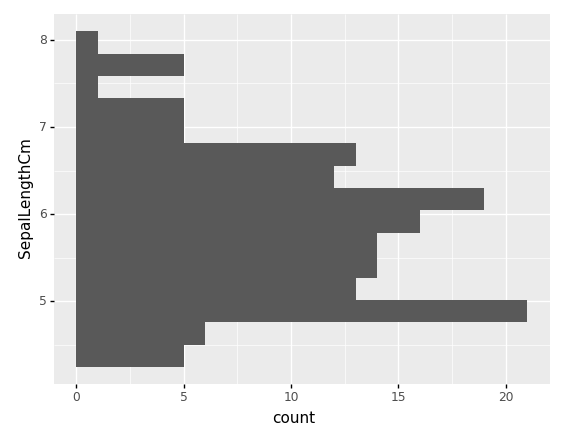### Themes

Themes are used for improving the looks of the data visualization. Plotnine includes a lot of theme which can be found in the plotnine’s themes API. Let’s use the above example with facets and try to make the visualization more interactive.

## Python3

 `import` `pandas as pd``from` `plotnine ``import` `ggplot, aes, facet_grid, labs, geom_col, theme_xkcd`` ` `# reading dataset``df ``=` `pd.read_csv(``"tips.csv"``)`` ` `(``    ``ggplot(df)``    ``+` `facet_grid(facets``=``"~sex"``)``    ``+` `aes(x``=``"day"``, y``=``"total_bill"``)``    ``+` `labs(``        ``x``=``"day"``,``        ``y``=``"total_bill"``,``    ``)``    ``+` `geom_col()``    ``+` `theme_xkcd()``)`

Output: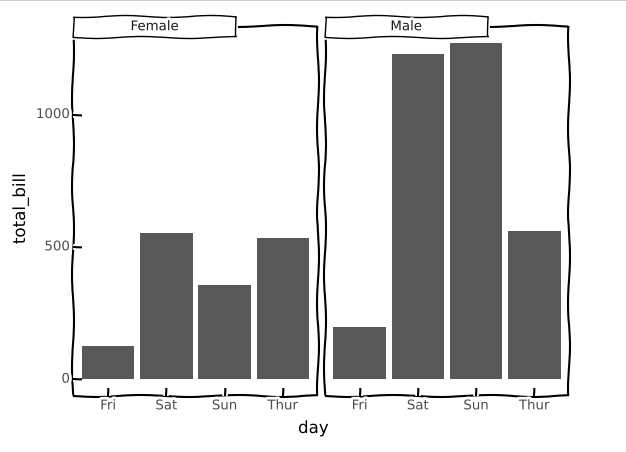We can also fill the color according to add more information to this graph. We can add color for the time variable in the above graph using the fill parameter of the aes function.

## Plotting Multidimensional Data

Till now we have seen how to plot more than 2 variables in the case of facets. Now let’s suppose we want to plot data using four variables, doing this with facets can be a little bit of hectic, but with using the color we can plot 4 variables in the same plot only. We can fill the color using the fill parameter of the aes() function.

## Python3

 `import` `pandas as pd``from` `plotnine ``import` `ggplot, aes, facet_grid, labs, geom_col, theme_xkcd`` ` `# reading dataset``df ``=` `pd.read_csv(``"tips.csv"``)`` ` `(``    ``ggplot(df)``    ``+` `facet_grid(facets``=``"~sex"``)``    ``+` `aes(x``=``"day"``, y``=``"total_bill"``, fill``=``"time"``)``    ``+` `labs(``        ``x``=``"day"``,``        ``y``=``"total_bill"``,``    ``)``    ``+` `geom_col()``    ``+` `theme_xkcd()``)`

Output: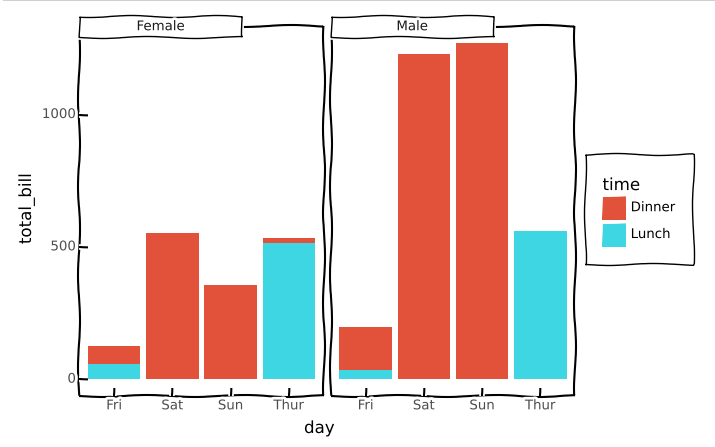## Saving the Plot

We can simply save the plot using the save() method. This method will esport the plot as an image.

## Python3

 `import` `pandas as pd``from` `plotnine ``import` `ggplot, aes, facet_grid, labs, geom_col, theme_xkcd`` ` `# reading dataset``df ``=` `pd.read_csv(``"tips.csv"``)`` ` `plot ``=` `(``    ``ggplot(df)``    ``+` `facet_grid(facets``=``"~sex"``)``    ``+` `aes(x``=``"day"``, y``=``"total_bill"``, fill``=``"time"``)``    ``+` `labs(``        ``x``=``"day"``,``        ``y``=``"total_bill"``,``    ``)``    ``+` `geom_col()``    ``+` `theme_xkcd()``)`` ` `plot.save(``"gfg plotnine tutorial.png"``)`

Output: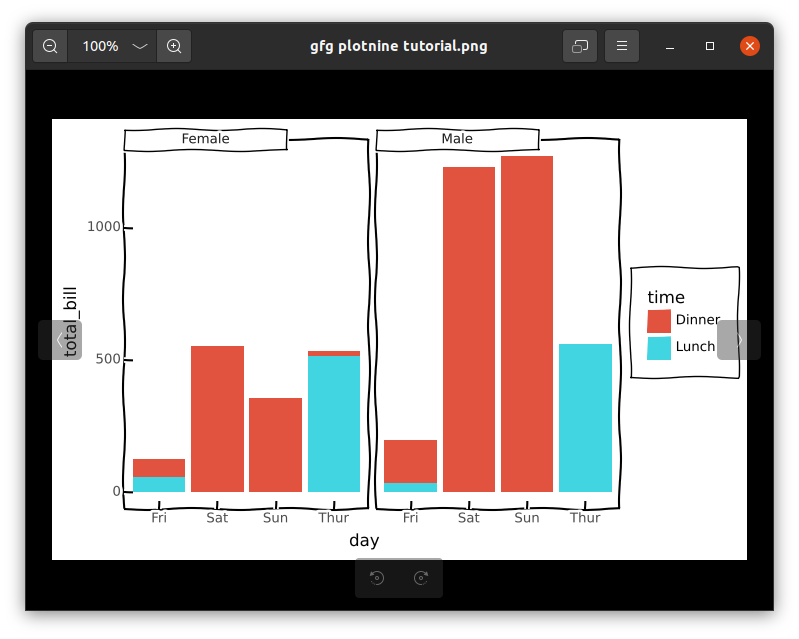My Personal Notes arrow_drop_up